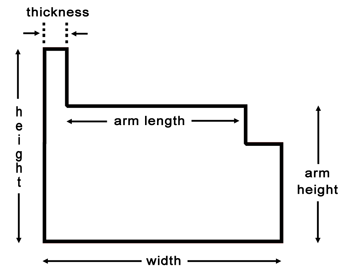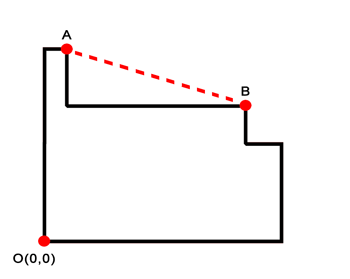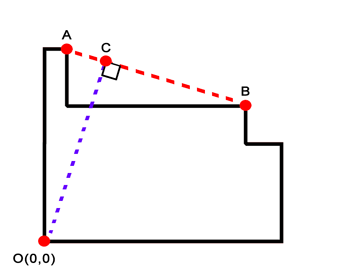Angled Width of a Sofa
View the Lesson | MATHguide homepage Updated July 7th, 2022

Problem: Find the angled width of the sofa with the given dimensions.Dimensions
height = 30 in
width = 36 in
arm length = 32 in
arm height = 28 in
thickness = 4 inLocation of Points
A(,)
B(,)

Slope of Segment ABEquation of Line AB
 y = x +Slope of Segment COEquation of Line CO
 y = xLocation of C
C(,)

Length of Segment CO
in